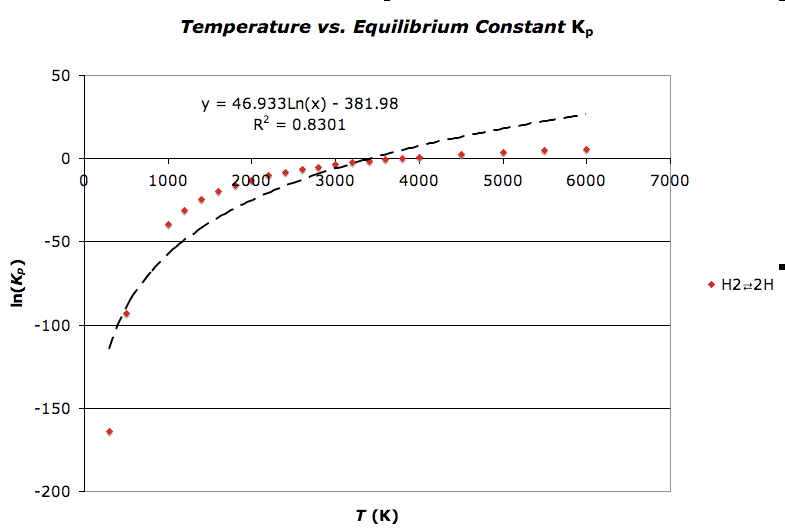ExcelGetting a Better Curve fit?

Hey folks,

Am I doing something wrong here? It just seems like I should be able to get a better fit. The data is plotted for the H2-->2H reaction data. I have attached the .xls file. Don't worry, no macrosI created it on a Mac, but hopefully everything converts correctly.

Here's a snapshot:Attachments

• EQ_Constants.xls
54 KB · Views: 174

Am I missing something very simple here? The title on your graph says "Temp versus K" but your axes are actually log(K) versus T.

Am I missing something very simple here? The title on your graph says "Temp versus K" but your axes are actually log(K) versus T.

No, you are not missing anythingIt is indeed log(K) and T. I have been messing around with the data, but forgot to change the title.

Good catch!

Are you sure there's a logarithmic dependency in your data set? If there was, then rescaling the x-axis to a log scale should give you a straight-line plot.

Hi,

I used my company's http://www.miraibio.com/masterplex-readerfit/curve-fitting-for-plate-readers.html" and used the Best Fit feature (and optimized for root mean square error) to find the best model equation and it appears to fit the 4 Parameter Logistic or 4PL surprisingly well:

I just realized that your x-axis was not in log scale. Here is the same curve fit with the x-axis on a linear scale.

Here are the parameters that were calculated:

A = -1862.64705 (lower asymptote)
B = 1.04715 (Hill slope)
C = 34.42647 (inflection point)
D = 13.67627 (upper asymptote)

R-square = 0.9999544 (although R-square is not really a really good indicator of nonlinear curves I thought I would post it anyway since you have it in your post)
RMSE = 0.28340 (root mean square error is more reliable and it is really good in your case)

Although MasterPlex ReaderFit is intended for ELISA analysis, it's application is flexible which is why I gave it a shot in your case. In all honesty, I was surprised at how nicely the curve fit came out to be (and I am assisting customers all day with curve fitting)!

Anyway, I hope this information helpsLast edited by a moderator:
You might consider:

Ln(Kp) = a / (c * Temp + d)^b + Offset

a = -1.4895890519700544E+04
b = 9.6571085998524553E-01
c = 3.3096509207808700E-01
d = -1.4626098473438096E+00
Offset = 1.5342375844418289E+01

as it fits with RMSE of 0.0061 and r-squared of 0.99999997, the fitting page is at:

http://zunzun.com/Equation/2/Miscellaneous/Polytrope%20Transform%20With%20Offset/ [Broken]

It appears to interpolate and extrapolate smoothly and reasonably:

[PLAIN]http://zunzun.com/images/temp1.png [Broken]

I found this using the http://zunzun.com "Function Finder" at:

http://zunzun.com/FunctionFinder/2/ [Broken]

and turning off the Polyfunctionals as I wanted named equations only.

James Phillips
http://zunzun.com
zunzun@zunzun.com

Last edited by a moderator:
This function might be better since it has only three coefficients, and the terms are simple functions.
RMSE = 0.0083, R-squared: 0.99999995,

y = a( Temp(K) ) + b( 1/Temp(K) ) + c( ln(Temp(K)) )

a = -2.0624423802678490E-04
b = -5.1874919532864478E+04
c = 1.7768380294902535E+00

James Phillips
http://zunzun.com
zunzun@zunzun.com

[PLAIN]http://zunzun.com/images/temp1.png [Broken]

Last edited by a moderator:
Hello everyone, I am facing a similar problem,

I have two curves, (assume data and model)

data is derived from measurements taken by an Ellipsometry instrument,curve.

model is derived from many many complex number calculations in excel,

as you can see in the picture attached, they fit, but not that well,
I have about 6 parameters that I can vary, all withing their certain limits.

How do I implement this so as to get the set of values to get the best fit possible ?

Any help would be greatly appreciated.

I cannot share with you the xls file due to the confidential nature of the data.
Let me know if you can help with the information I have already provided.

Thanks.

Attachments

The program peakfit might help: http://www.sigmaplot.com/products/peakfit/peakfit.php [Broken]

James

Last edited by a moderator:
Thank you for your quick response.

Is it possible to do so in excel ?

Is it possible to do so in excel ?

Not that I know of.

James

Thank you for your quick response.

Is it possible to do so in excel ?

I think that the only way to something like this in excel is if you can guess the form that the function takes (which can be quite difficult). For example, you could guess that your funtion takes the form y = A*x^2 + B*x + C (which, of course, it does not) and then use your x values, along with some assumed values of A,B,C, to generate a set of corresponding y values.

Now you can have A,B, and C located in some cells, say A1,A2,A3. And you would also have your original y data in a column and your new guessed function's y data in another. You can quantify the overall error between the original and new using the RSME function. Place that in a cell some where, say B1.

Now use the Excel solver function. This allows you to minimize a value in one cell by adjusting values in other cells. You would use this to minimize the RMSE in cell B1 by adjusting the values of the constants in A1,A2 and A3.

Again, this all hinges on you being able to guess an appropriate form of the function that fits your data, which I do not think I can help you with.

DrDu